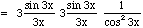Date: Tue, 16 Sep 1997 10:21:38 -0600 (CST)
To: QandQ@MathCentral.uregina.ca
Subject: a limit problem

Name: Brian Ray

Question:
what is the limit, as x approaches 0, or tan^23x/x^2? (read, tan squared 3x over...)

Hi Brian,
Many of these trigonometric limits can be evaluated by relating them to the fact that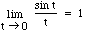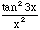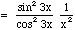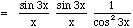To relate each of the first two fractions to the known limit above we need the quantity inside the sine function, ie 3x to be the same as the quantity in the denominator. Thus multiply the numerator and denominator in each of the first two fractions by 3 to get